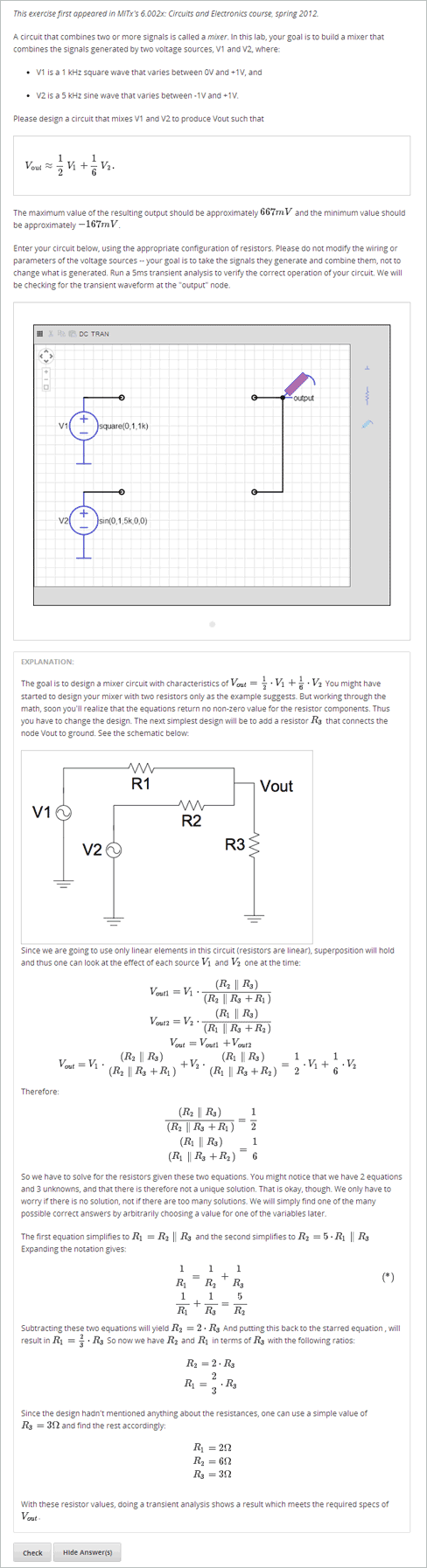# 8.5. Circuit Schematic Builder Problem¶

In circuit schematic builder problems, students can arrange circuit elements such as voltage sources, capacitors, resistors, and MOSFETs on an interactive grid. They then submit a DC, AC, or transient analysis of their circuit to the system for grading.## 8.5.1. Create a Circuit Schematic Builder Problem¶

1. In the unit where you want to create the problem, click Problem under Add New Component, and then click the Advanced tab.
2. Click Circuit Schematic Builder.
3. In the component that appears, click Edit.
4. In the component editor, replace the example code with your own code.
5. Click Save.

Problem Code

To create the problem in the image above, paste the following code into the Advanced Editor.

<problem>
<p>Make a voltage divider that splits the provided voltage evenly.</p>
<schematicresponse>
<center>
<schematic height="500" width="600" parts="g,r" analyses="dc"
initial_value="[["v",[168,144,0],{"value":"dc(1)","_json_":0},["1","0"]],["r",[296,120,0],{"r":"1","_json_":1},["1","output"]],["L",[296,168,3],{"label":"output","_json_":2},["output"]],["w",[296,216,168,216]],["w",[168,216,168,192]],["w",[168,144,168,120]],["w",[168,120,296,120]],["g",[168,216,0],{"_json_":7},["0"]],["view",-67.49999999999994,-78.49999999999994,1.6000000000000003,"50","10","1G",null,"100","1","1000"]]"
/>
</center>
for response in submission:
if response == 'dc':
for node in response[1:]:
dc_value = node['output']
if dc_value == .5:
correct = ['correct']
else:
correct = ['incorrect']
</schematicresponse>
<schematicresponse>
<p>Make a high pass filter.</p>
<center>
<schematic height="500" width="600" parts="g,r,s,c" analyses="ac"
submit_analyses="{"ac":[["NodeA",1,9]]}"
initial_value="[["v",[160,152,0],{"name":"v1","value":"sin(0,1,1,0,0)","_json_":0},["1","0"]],["w",[160,200,240,200]],["g",[160,200,0],{"_json_":2},["0"]],["L",[240,152,3],{"label":"NodeA","_json_":3},["NodeA"]],["s",[240,152,0],{"color":"cyan","offset":"0","_json_":4},["NodeA"]],["view",64.55878906250004,54.114697265625054,2.5000000000000004,"50","10","1G",null,"100","1","1000"]]"/>
</center>
ac_values = None
for response in submission:
if response == 'ac':
for node in response[1:]:
ac_values = node['NodeA']
print "the ac analysis value:", ac_values
if ac_values == None:
correct = ['incorrect']
elif ac_values < ac_values:
correct = ['correct']
else:
correct = ['incorrect']